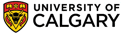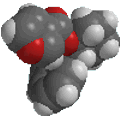Chapter 7 : StereochemistryMeso compounds
 In the simplest case, a compound with two chirality centers where there is the same set of four groups at each chirality center, the combination where the four groups are arranged such that the centers are mirror images of each other (i.e. where the molecule has an internal mirror plane) is a meso compound. The grey atoms are the chirality centers. Each grey atom is attached to one green, one maroon, one purple and one grey. The left side is the mirror image of the right side.

For example consider 2,3-dichlorobutane.  There are two chirality centers (C2 and C3), each with -H, -Cl, -CH3 and the other -CHClCH3 groups attached. Since there are two chirality centers, then, at least in principle, there are 4 possible permutations of the stereocenters : (R,R), (R,S), (S,R) and (S,S)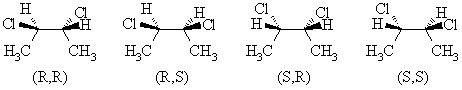The (R,R) and (S,S) structures are non-superimposable mirror images, so they are a pair of enantiomers.
If we look at the (R,S) and (S,R) as drawn above, it should be reasonably easy to recognise that these two structures are actually the same thing because they are superimposable, i.e. (R,S) º (S,R). This structure is the meso isomer. To verify this, rotate one of the models 180 degrees about the vertical axis to make it look the same as the other structure.
In the conformations of the (R,S) shown above, the mirror plane should be obvious (vertical plane bisecting the middle of the central C-C bond).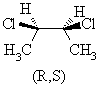(R,S)-2,3-dichlorobutane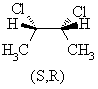(S,R)-2,3-dichlorobutane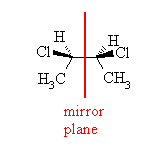However, let's look at the other important conformation of the meso isomer and make sure we can recognise it is the meso isomer.The relationship of A to B is not immediately obvious. However, once the right hand end has been rotated about the central C-C bond by 180 degrees, A can been seen to be the mirror image of B .  A quick way of recognising whether a molecule is achiral is to look for a plane of symmetry.
 Important properties of meso compunds that you should know:  For simple meso compounds with 2 chirality centers :  they are optically inactive they must be (R,S) configuration they are diastereomers of the (R,R) and (S,S) isomers© Dr. Ian Hunt, Department of Chemistry